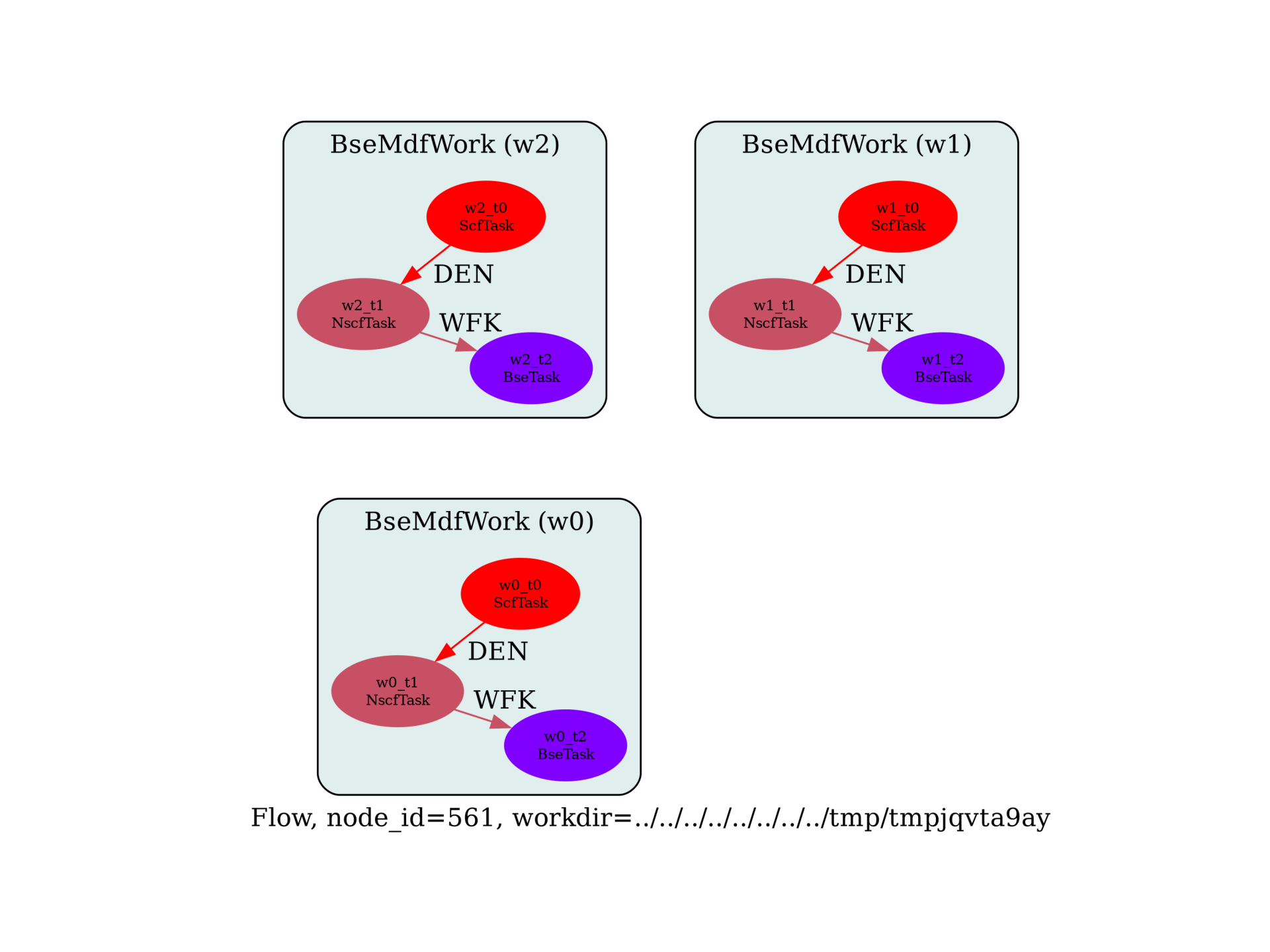# Raman Flow with BSE and frozen phonon¶

This script shows how to perform a Raman calculation with excitonic effects included with the BSE formalism.

import sys
import os
import numpy as np
import abipy.abilab as abilab
import abipy.data as abidata
from abipy import flowtk

def build_flow(options):
# Working directory (default is the name of the script with '.py' removed and "run_" replaced by "flow_")
if not options.workdir:
options.workdir = os.path.basename(sys.argv).replace(".py", "").replace("run_","flow_")

flow = flowtk.Flow(options.workdir, manager=options.manager)

pseudos = abidata.pseudos("14si.pspnc")

# Get the unperturbed structure.
base_structure = abidata.structure_from_ucell("Si")

etas = [-.001, 0, +.001]
ph_displ = np.reshape(np.zeros(3*len(base_structure)), (-1,3))
ph_displ[0,:] = [+1, 0, 0]
ph_displ[1,:] = [-1, 0, 0]

# Build new structures by displacing atoms according to the phonon displacement
# ph_displ (in cartesian coordinates). The Displacement is normalized so that
# the maximum atomic diplacement is 1 Angstrom and then multiplied by eta.
modifier = abilab.StructureModifier(base_structure)

displaced_structures = modifier.displace(ph_displ, etas, frac_coords=False)

# Generate the different shifts to average
ndiv = 2
shift1D = np.arange(1,2*ndiv+1,2)/(2*ndiv)
all_shifts = [[x,y,z] for x in shift1D for y in shift1D for z in shift1D]
all_shifts = [[0, 0, 0]]

for structure, eta in zip(displaced_structures, etas):
for shift in all_shifts:
flow.register_work(raman_work(structure, pseudos, shift))

return flow

def raman_work(structure, pseudos, shiftk, paral_kgb=1):
# Generate 3 different input files for computing optical properties with BSE.

# Global variables
global_vars = dict(
ecut=8,
istwfk="*1",
chksymbreak=0,
#nstep=4,
nstep=10,
paral_kgb=paral_kgb,
)

# GS run
scf_inp = abilab.AbinitInput(structure, pseudos=pseudos)
scf_inp.set_vars(global_vars)
scf_inp.set_kmesh(ngkpt=[2, 2, 2], shiftk=shiftk)
scf_inp["tolvrs"] = 1e-6

# NSCF run
nscf_inp = abilab.AbinitInput(structure, pseudos=pseudos)
nscf_inp.set_vars(global_vars)
nscf_inp.set_kmesh(ngkpt=[2, 2, 2], shiftk=shiftk)

nscf_inp.set_vars(
tolwfr=1e-8,
nband=12,
nbdbuf=4,
iscf=-2,
)

# BSE run with Model dielectric function and Haydock (only resonant + W + v)
# Note that SCR file is not needed here
bse_inp = abilab.AbinitInput(structure, pseudos=pseudos)
bse_inp.set_vars(global_vars)
bse_inp.set_kmesh(ngkpt=[2, 2, 2], shiftk=shiftk)

bse_inp.set_vars(
optdriver=99,
ecutwfn=global_vars["ecut"],
ecuteps=3,
inclvkb=2,
bs_algorithm=2,       # Haydock
bs_haydock_niter=4,   # No. of iterations for Haydock
bs_exchange_term=1,
bs_coulomb_term=21,   # Use model W and full W_GG.
mdf_epsinf=12.0,
bs_calctype=1,        # Use KS energies and orbitals to construct L0
mbpt_sciss="0.8 eV",
bs_coupling=0,
bs_loband=2,
nband=6,
#bs_freq_mesh="0 10 0.1 eV",
bs_hayd_term=0,      # No terminator
)

# Build the work representing a BSE run with model dielectric function.
return flowtk.BseMdfWork(scf_inp, nscf_inp, bse_inp)

# This block generates the thumbnails in the AbiPy gallery.
# You can safely REMOVE this part if you are using this script for production runs.
__name__ = None
import tempfile
options = flowtk.build_flow_main_parser().parse_args(["-w", tempfile.mkdtemp()])
build_flow(options).graphviz_imshow()

@flowtk.flow_main
def main(options):
"""
This is our main function that will be invoked by the script.
flow_main is a decorator implementing the command line interface.
Command line args are stored in options.
"""
return build_flow(options)

if __name__ == "__main__":
sys.exit(main())Out:

/Users/gmatteo/git_repos/pymatgen/pymatgen/util/plotting.py:550: UserWarning: Matplotlib is currently using agg, which is a non-GUI backend, so cannot show the figure.
plt.show()


Run the script with:

run_raman_bse.py -s

then use:

abirun.py flow_raman_bse robot MDF

to analyze all the macroscopic dielectric functions produced by the Flow with the robot. Inside the ipython terminal type:

 %matplotlib
 robot.plot()


to compare the real and imaginary part of the macroscopic dielectric function for the different displacements.Total running time of the script: ( 0 minutes 0.833 seconds)

Gallery generated by Sphinx-Gallery